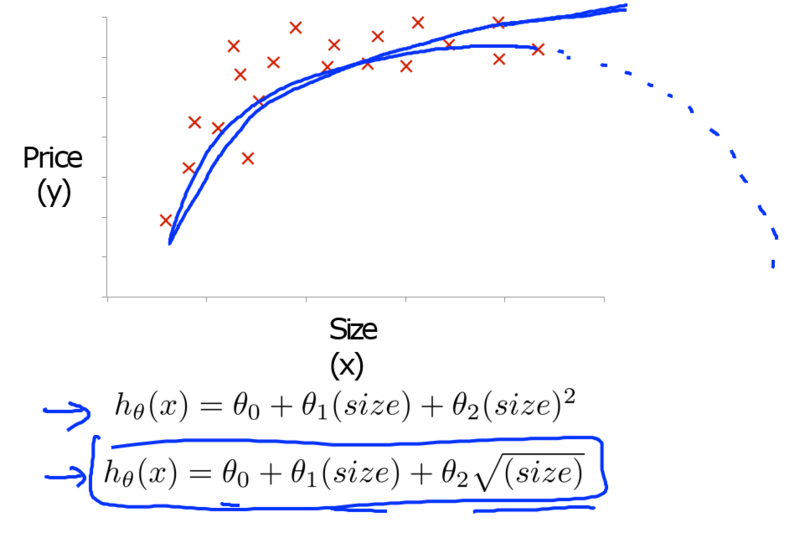## 假设函数

h_\theta(x) = \theta_0+\theta_1x_1+\theta_2x_2+\theta_3x_3+…+\theta_nx_n

h_\theta(x) = \begin{bmatrix} \theta_0&\theta_1&…&\theta_n \end{bmatrix} \begin{bmatrix} x_0\\x_1\\x_2\\…\\x_n \end{bmatrix} = = \theta^Tx

## 梯度下降

repeat until convergence:{
\theta_0 := \theta_0 – \alpha \frac{1}{m}\sum_{i=1}^{m}(h_\theta(x^(i))-y^(i))x_0^(i)

\theta_1 := \theta_1 – \alpha \frac{1}{m}\sum_{i=1}^{m}(h_\theta(x^(i))-y^(i))x_1^(i)

\theta_2 := \theta_2 – \alpha \frac{1}{m}\sum_{i=1}^{m}(h_\theta(x^(i))-y^(i))x_2^(i)

\theta_j := \theta_j – \alpha\frac{1}{m}\sum_{i=1}^{m}(h_\theta(x^(i))-y^(i))x_j^(i)\quad j:=0,1…n
}

## 特征缩放

x_1 = 房子面积（0-200平米）\
x_2 = 房间数量（1-5）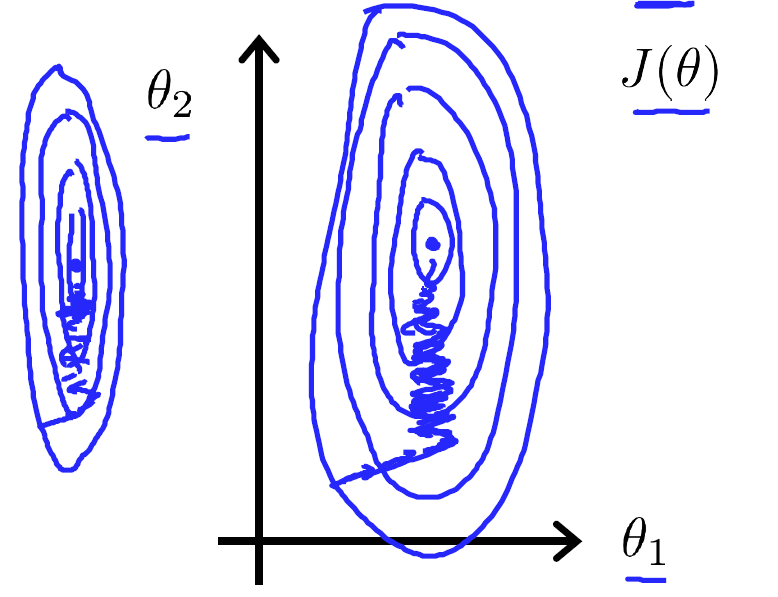x_i := \frac{x_i-\mu_i}{s_i}

x_1 := \frac{110-平均面积(假设为100)}{200} = 0.05\
x_2 := \frac{3-平均每户房子包含的房间数（假设为2）}{4} = 0.25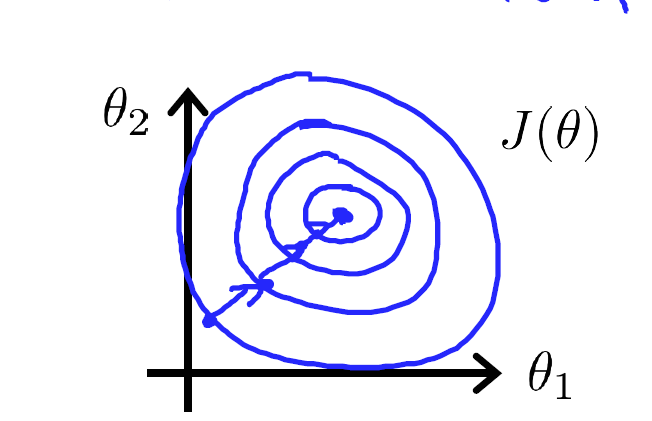## 学习速率

\theta_j := \theta_j – \frac{\partial}{\partial\theta_j}J(\theta)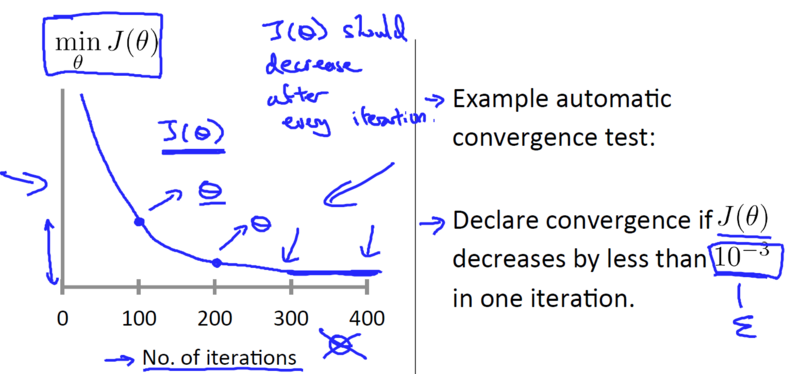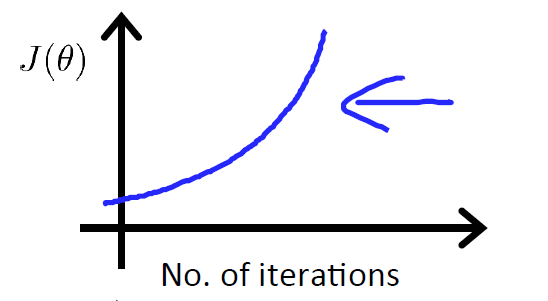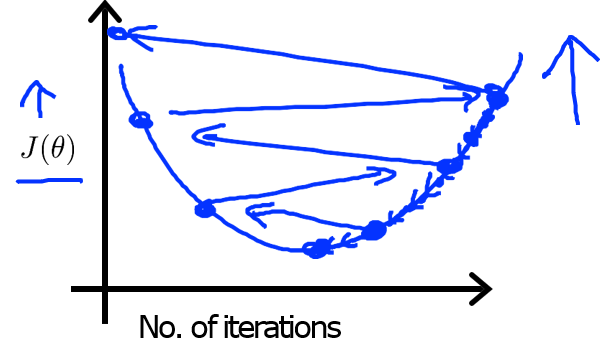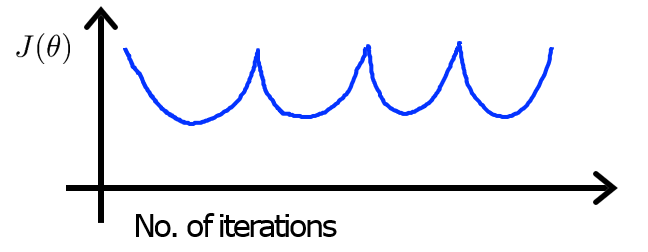## 多项式回归

h_\theta(x) = \theta_0+\theta_1x_1+\theta_2x_1^2+\theta_3x_1^3